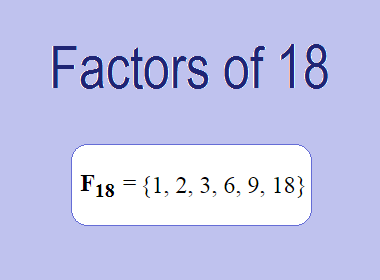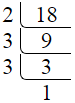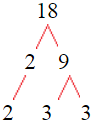# Factors of 18The factors of 18 are 1, 2, 3, 6, 9, and 18 i.e. F18 = {1, 2, 3, 6, 9, 18}. The factors of 18 are all the numbers that can divide 18 without leaving a remainder.

We can check if these numbers are factors of 18 by dividing 18 by each of them. If the result is a whole number, then the number is a factor of 18. Let's do this for each of the numbers listed above:

·        1 is a factor of 18 because 18 divided by 1 is 18.

·        2 is a factor of 18 because 18 divided by 2 is 9.

·        3 is a factor of 18 because 18 divided by 3 is 6.

·        6 is a factor of 18 because 18 divided by 6 is 3.

·        9 is a factor of 18 because 18 divided by 9 is 2.

·        18 is a factor of 18 because 18 divided by 18 is 1.

## How to Find Factors of 18?

1 and the number itself are the factors of every number. So, 1 and 18 are two factors of 18. To find the other factors of 18, we can start by dividing 18 by the numbers between 1 and 18. If we divide 18 by 2, we get a remainder of 0. Therefore, 2 is a factor of 18. If we divide 18 by 3, we get a remainder of 0. Therefore, 3 is also a factor of 18.

Next, we can check if 4 is a factor of 18. If we divide 18 by 4, we get a remainder of 2. Therefore, 4 is not a factor of 18. We can continue this process for all the possible factors of 18.

Through this process, we can find that the factors of 18 are 1, 2, 3, 6, 9, and 18. These are the only numbers that can divide 18 without leaving a remainder.

********************

********************

## Properties of the Factors of 18

The factors of 18 have some interesting properties. One of the properties is that the sum of the factors of 18 is equal to 39. We can see this by adding all the factors of 18 together:

1 + 2 + 3 + 6 + 9 + 18 = 39

Another property of the factors of 18 is that the prime factors of 18 are 2, and 3 only.

## Applications of the Factors of 18

The factors of 18 have several applications in mathematics. One of the applications is in finding the highest common factor (HCF) of two or more numbers. The HCF is the largest factor that two or more numbers have in common. For example, to find the HCF of 18 and 24, we need to find the factors of both numbers and identify the largest factor they have in common. The factors of 18 are 1, 2, 3, 6, 9, and 18. The factors of 24 are 1, 2, 3, 4, 6, 8, 12, and 24. The largest factor that they have in common is 6. Therefore, the HCF of 18 and 24 is 6.

Another application of the factors of 18 is in prime factorization. Prime factorization is the process of expressing a number as the product of its prime factors. The prime factors of 18 are 2, and 3 since these are the only prime numbers that can divide 18 without leaving a remainder. Therefore, we can express 18 as:

18 = 2 × 3 × 3

We can do prime factorization by division and factor tree method also. Here is the prime factorization of 18 by division method,18 = 2 × 3 × 3

Here is the prime factorization of 18 by the factor tree method,18 = 2 × 3 × 3

## Conclusion

The factors of 18 are the numbers that can divide 18 without leaving a remainder. The factors of 18 are 1, 2, 3, 6, 9, and 18. The factors of 18 have some interesting properties, such as having a sum of 39. The factors of 18 have several applications in mathematics, such as finding the highest common factor and prime factorization.